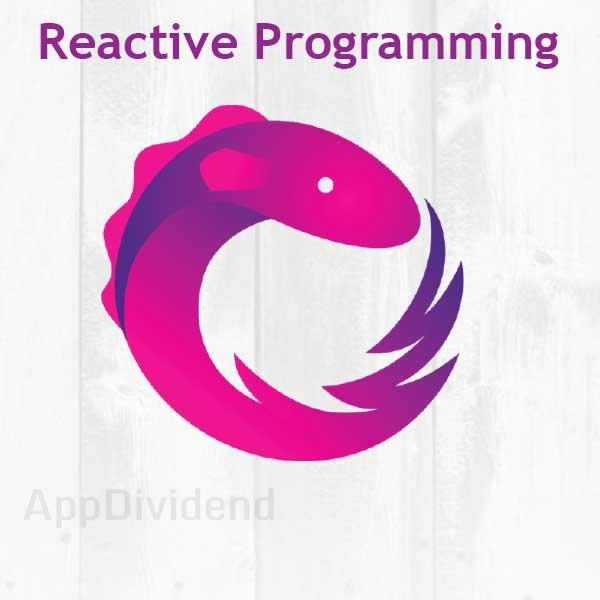What is Reactive Programming in JavascriptHey web developers, In today’s AppDividend Tutorial, I have briefly described What is Reactive Programming in Javascript?

If you are taking this article, then Node.js is installed on your machine. It is a basic necessity.

It’s time to rethink the basic Software Architecture because Conventional Programming is not satisfied today’s  modern software application requirement.

Reactive Programming

It is a programming of event streams happens in time. Reactive Programming is like a sequence of events occur in time. It is an Asynchronous Programming concept around data streams. There are two types of data streams.

1. Static Data Streams (Arrays)
2. Dynamic Data Streams (Event Emitters)

In general programming paradigm if z = x + y at the time of declaration then z will be the sum of x and y, but after that statement, if we change the value of x and y then z will be unchanged. So no effects after that sum statement.

Example # 1

``````//main.js

var x = 10;
var y = 20;
let z = x + y;
console.log(z);
x = 100;
y = 200;
console.log(z);``````

Possible Errors

1. If you perform code directly in your browser, then chances are very high to fail the webpack compilation process.

Possible Solutions

1.  Beginner’s Guide To Setup ES6 Development Environment  Follow this article strictly and put above code in the main.js file

In both console report, we will get the same thing, and that is 30 and 30

The value of x and y does not effect in variable z, so in Imperative Programming, The concept of Dynamic Change is missing.

Here in example, we are using ES6 so, we need to download ES6 version of RxJS

``npm install rxjs-es --save``
``````import {Observable} from 'rxjs-es';
let streamA = Observable.of(3);
let streamB = streamA.map(a => 3 * a);
streamB.subscribe(b => console.log(b));``````

In the console, we can see the output of 9

Now we need to specify the Dynamic Behaviour of the Streams at the Declaration of the time and not after that otherwise It will not work. So dynamic code will look like this.

``````import {Observable} from 'rxjs-es';
let streamA = Observable.of(3, 4); // At the time of declaration, we need to assign the dynamic behaviour.
let streamB = streamA.map(a => 10 * a);
streamB.subscribe(b => console.log(b));``````

Now your new output will be 9 and 12

In Reactive Programming, everything is Streams that occur in time. A stream is a progression(sequence) of ongoing events specific to time.

Example # 2

``````//main.js

let a = [1,2,3,4,5,6,7,8,9];
console.log(a); // [1,2,3,4,5,6,7,8,9]``````

It logs the array of the values, and it does not relate to kind any of time interference.

``````//main.js

var a = [1,2,3,4,5,6,7,8,9];
var b = a.filter(x => x > 4)
.reduce((x,y) => x + y);
console.log(b);``````

Run again, you will see in console 35.

Now above example is simple, we have just taken an array and then filter with some condition and then reduce it to the previous and next value to get the final value of b.

This article uses environment setup of  Beginner’s Guide To Setup ES6 Development Environment this article. So, still, do not know then please check out that article and you will up and running. Now we need to add RxJS into the step forward.

Step 1: Check your package.json file, It will look like this.

``````1
3
5
7
9
11``````

In above code, the values are logged at some time interval in our case 500 milliseconds, which is Asynchronous Paradigm.

When interval event is fire, we got the logged values in our console, and It is awesome right!!

We got the event streams at particular time, and we can also apply map, filter, reduce and other array functions to the events streams

``````//main.js

import {Observable} from 'rxjs-es';

let output = Observable.interval(500)
.take(6)
.map(i => [1,3,5,7,9,11][i]);
let result = output.map(x => x)
.filter(x => x > 5)
.reduce((x, y) => x + y);
result.subscribe(x => console.log(x));``````

And we get 27 in the console.

Memorable Points

1. Reactive Programming allows you to set Dynamic Behaviour only at Declaration Time.
2. Reactive Programming is different from Imperative programming because as the name suggests it reacts when something in our application is occurring or done.
3. It relies on Asynchronous Nature that happens in time.
4. It stays responsive in the case of Failure and Success.
5. In the Reactive world, we can not wait on something happen to the program, we need to continue the execution, and if something happens, we need to React to it. That is what Async Pattern is.

If you still have any doubt then ask in a comment below, I am happy to help you out.